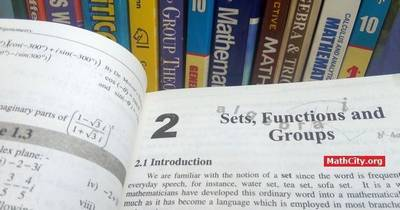# Chapter 02: Sets, Functions and Groups

Notes (Solutions) of Chapter 02: Sets, Functions and Groups, Text Book of Algebra and Trigonometry Class XI (Mathematics FSc Part 1 or HSSC-I), Punjab Text Book Board, Lahore.• Introduction
• Exercise 2.1
• Operations on Sets
• Venn Diagrams
• Exercise 2.2
• Operations on Three Sets
• Properties of Union and Intersection
• Exercise 2.3
• Inductive and Deductive Logic
• Aristotelian and non-Aristotelian logics
• Symbolic Logic
• Implication or Conditional
• Bicopnditional : $p\leftrightarrow q$
• Conditionals related with a given Conditional.
• Tautologies
• Quantifiers
• Exercise 2.4
• Truth Seats, A Link between Set Theory and Logic
• Exercise 2.5
• Relations
• Functions
• Inverse of a Function
• Exercise 2.6
• Binary Operations
• Properties of Binary Operations
• Exercise 2.7
• Groups
• Solution of Linear Equations
• Reversal Law of Inverses
• Exercise 2.8
• Exercise 2.2, Question 4, Part iii, the answer is wrong. The correct answer is: A U B = {1,2,3,4,5,6,8,10} — Ubaid ur Rehman (GC Attock) 2016/01/19 06:10

The following short questions of this chapter was send by Mr. Akhtar Abbas.

• fsc/fsc_part_1_solutions/ch02